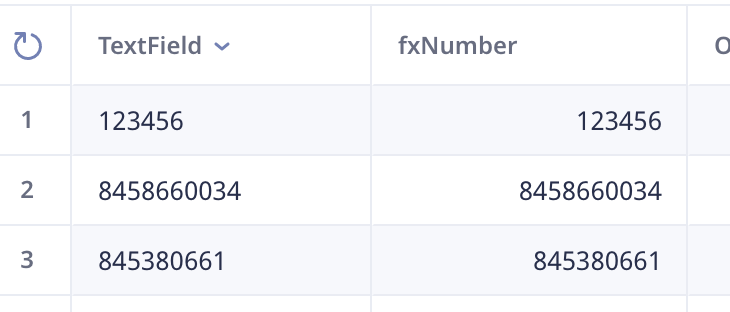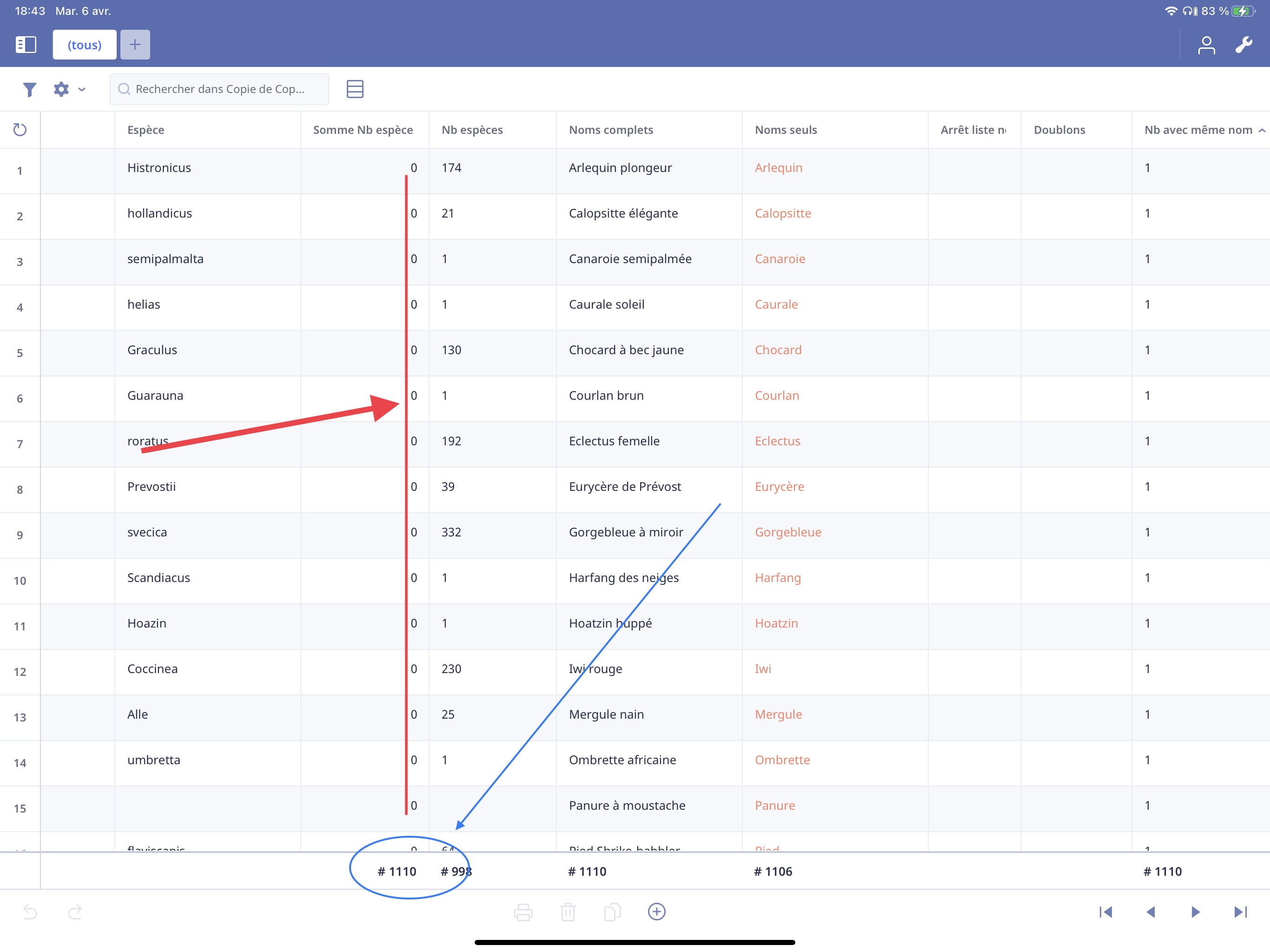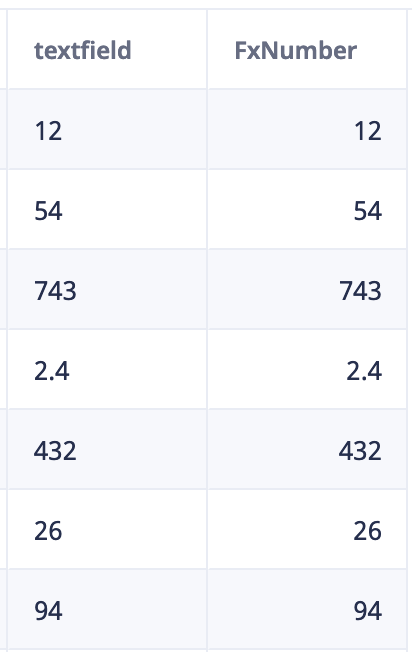### A formula to really change numbers in text in a column in a real numbers columns ?

Hi,

How can we change numbers in text into real numbers  ?

I used the formula :

format(number('Number of 'species'), "0000")

It works well but if I click on the filter box at the top of the column I don't have access to the usual tabs when it's on a number column :

sum -average -mini -maxi ( that are not in a colonne with numbers-text)

So I can't for example click on "sum"

Is there another formula that really transforms a "text number" column into a "real number" column so I could be able to access the functions as "sum" ?

Thank you

I added a forumal field

then pointed it a text field holding Text numbers

then added this formula

number(TextField)

hey presto as both shown in screen shot

and now i can sum etc on this new colThanks a lot Mel,

What do you mean by :

"I added a forumal field then pointed it a text field holding Text numbers

then added this formula"

I guess "forumal" field links to "formula" field

But what about  "pointed it a text field holding Text numbers" ?

Vould you give an example ?

(I am French)

It's ok Mel. It works very well

Thanks again.

ha ha spelling mistake

i meant formula field !! :-)

I have tried once your formula and, as I said, it worked fine.

I tried it again with another base and the formula column is now full of zero but the sum seems still to work…What I do :

I create a formula field

I enter this formula :

number(TextField)

Ex:

number (Column7)

-I Go to the funnel and drag and drop the formula field from right to left to make it displayed in the table

-I Click on the top of the column and click on "Sum"

>At the bottom of the column the total is displayed (addition of the values and not the ones of the rows)

From you picture it looks like you selected Count not Sum. You see the # next to 1110. That is the count symbol. ∑ is the sum symbol.

Are you try trying to convert the "Nb especes" field to a number and they show up as 0?

This is my example. When the numbers are aligned on the left side they are being treated as text. When numbers are aligned on the right side they are being treated as numbers.Hi Fred

"Are you try trying to convert the "Nb especes" field to a number and they show up as 0 ?"

Yes and I don't understand why there are zeros and not numbers…

1 2
Reply